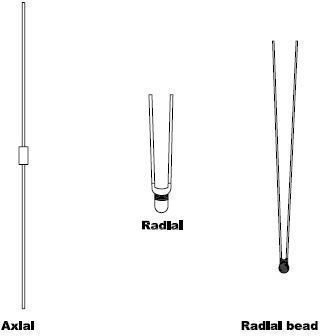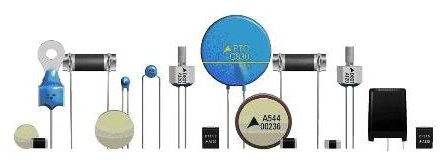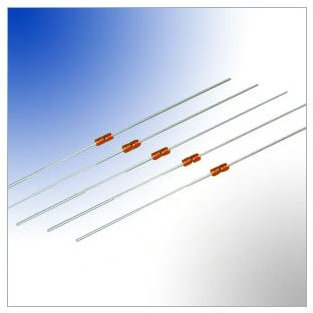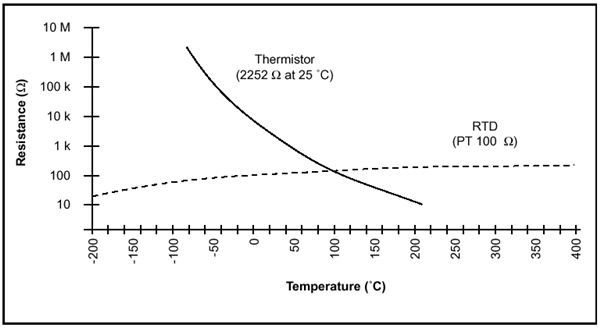# What are Thermistors? How Thermistors Work?

## What are Thermistors?

Thermistors are one of the most commonly used devices for the measurement of temperature. The thermistors are resistors whose resistance changes with the temperature. While for most of the metals the resistance increases with temperature, the thermistors respond negatively to the temperature and their resistance decreases with the increase in temperature. Since the resistance of thermistors is dependent on the temperature, they can be connected in the electrical circuit to measure the temperature of the body.

## Materials used for Thermistors and their Forms

The thermistors are made up of ceramic like semiconducting materials. They are mostly composed of oxides of manganese, nickel and cobalt having the resistivities if about 100 to 450,000 ohm-cm. Since the resistivity of the thermistors is very high the resistance of the circuit in which they are connected for measurement of temperature can be measured easily. This resistance is calibrated against, the input quantity, which is the temperature, and its value can be obtained easily.

Thermistors are available in various shapes like disc, rod, washer, bead etc. They are of small size and they all can be fitted easily to the body whose temperature has to be measured and also can be connected to the circuit easily. Most of the thermistors are quite cheap.

## Thermistor Shapes## Principle of Working of Thermistors

As mentioned earlier the resistance of the thermistors decreases with the increase its temperature. The resistance of thermistor is given by:

R = Ro ek

K = β(1/T – 1/To)

Where R is the resistance of the thermistor at any temperature T in oK (degree Kelvin)

Ro is the resistance of the thermistors at particular reference temperature Toin oK

e is the base of the Naperian logarithms

β is a constant whose value ranges from 3400 to 3900 depending on the material used for the thermistors and its composition.

The thermistor acts as the temperature sensor and it is placed on the body whose temperature is to be measured. It is also connected in the electric circuit. When the temperature of the body changes, the resistance of the thermistor also changes, which is indicated by the circuit directly as the temperature since resistance is calibrated against the temperature. The thermistor can also be used for some control which is dependent on the temperature.## Advantages of Thermistors

Here are some of the advantages of the thermistors

1) When the resistors are connected in the electrical circuit, heat is dissipated in the circuit due to flow of current. This heat tends to increase the temperature of the resistor due to which their resistance changes. For the thermistor the definite value of the resistance is reached at the given ambient conditions due to which the effect of this heat is reduced.

2) In certain cases even the ambient conditions keep on changing, this is compensated by the negative temperature characteristics of the thermistor. This is quite convenient against the materials that have positive resistance characteristics for the temperature.

3) The thermistors are used not only for the measurement of temperature, but also for the measurement of pressure, liquid level, power etc.

4) They are also used as the controls, overload protectors, giving warnings etc.

5) The size of the thermistors is very small and they are very low in cost. However, since their size is small they have to be operated at lower current levels.

## Reference

Book: Mechanical Measurements by Thomas G. Beckwith and N. Lewis Buck

## Images Courtesy

5) https://www.sselec.com/data/Thermistors.htm

6) https://www.spoerle.com/cda/distilive/0,,4476,00.html